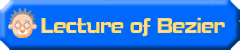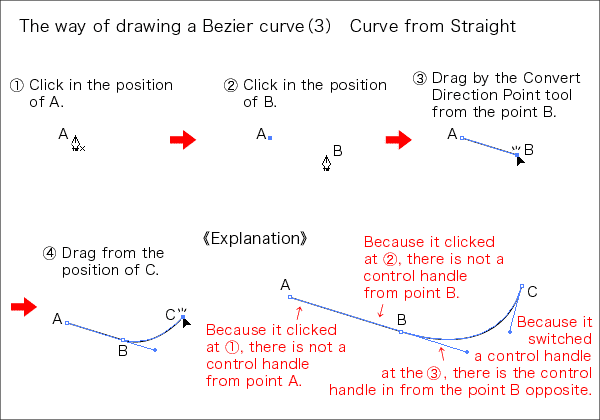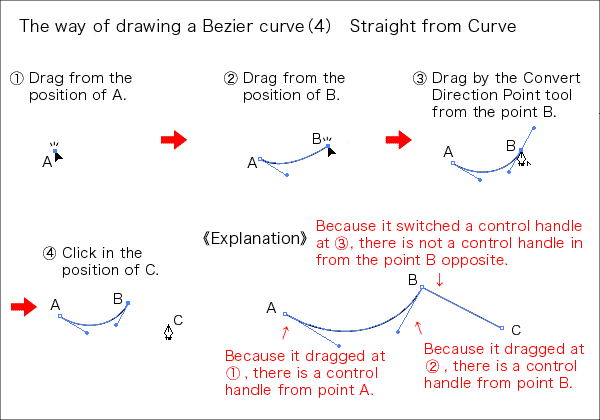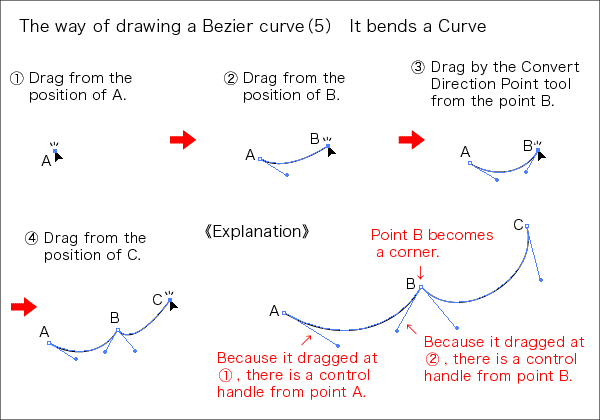### 6.It uses the Convert Direction Point tool. ( Ways 3, 4, 5 of drawing of the Bezier curve )

It uses a Convert Direction Point tool to change to the straight line from the curve or the curve from the straight line when drawing a Bezier curve in Adobe Illustrator.It may choose a Convert Direction Point tool from the toolbox of Adobe Illustrator but to be general is as the Pen tool and

It presses [option]key (Mac)/[Alt]key (Win)

and it changes to the Convert Direction Point tool.Being as the Convert Direction Point tool for a long time while pushing [option]/ [Alt ]key .It returns to the original Pen tool when letting the key go.

Way, in Adobe Illustrator, to repeat only, too, becomes a Convert Direction Point tool automatically with the end point in the path as the Pen tool.In the case like " the way 5 of drawing of the Bezier curve bending from the curve and being a curve " which comes out from behind, it doesn't work as the Convert Direction Point tool ( It knows that it attempts to draw a Bezier curve actually ).

Let's master that [option]key (Mac)/[Alt]key (Win) switches Pen tool to the Convert Direction Point tool beforehand by all means.

The 3rd pattern is a curve from the straight line.1)Because the path begins with the straight line ( there is not a control handle ), it clicks in the position of A.

2)Because it draws a straight line, it clicks in the B position.

3)Because it changes to the curve ( there is a control handle ), it drags by the Convert Direction Point tool from the point B.

4)Because it draws a curve ( there is a control handle ), it drags from the position of C.

When drawing a Bezier curve, the control handle comes out from the end point when dragging by the Convert Direction Point tool.Because there is a control handle, it switches over to the curve from here.Oppositely, it is the pattern which draws a straight line from the curve.1)Because the path begins with the curve ( there is a control handle ), it drags from the position of A.

2)Because it draws a curve , it drags from the B position.

3)Because it changes to the straight line ( there is not a control handle ), it clicks point B by the Convert Direction Point tool.

4)Because it draws a straight line ( there is not a control handle ), it clicks the position of C.

When clicking the end point, this time, the control handle passes away.Therefore, it switches over to the straight line from here.In the last, it resembles a curve from 2 curves but it is when bending the interval.1)Because the path begins with the curve ( there is a control handle ), it drags from the position of A.

2)Because it draws a curve, it drags from the B position.

3)It makes a curve from the curve but because it bends a path, it drags by the Convert Direction Point tool from the point B.

4)Because it draws a curve, it drags from the position of C.

When dragging by the Convert Direction Point tool from the end point, the direction can be changed by breaking one out of two control handles which are stretching, being contrary from the anchor points.When breaking a control handle, the anchor points becomes a corner.All paths can be made with this 5 corner combination.Be always conscious of whether the drawn part is a straight line or a curve and let's control a control handle.